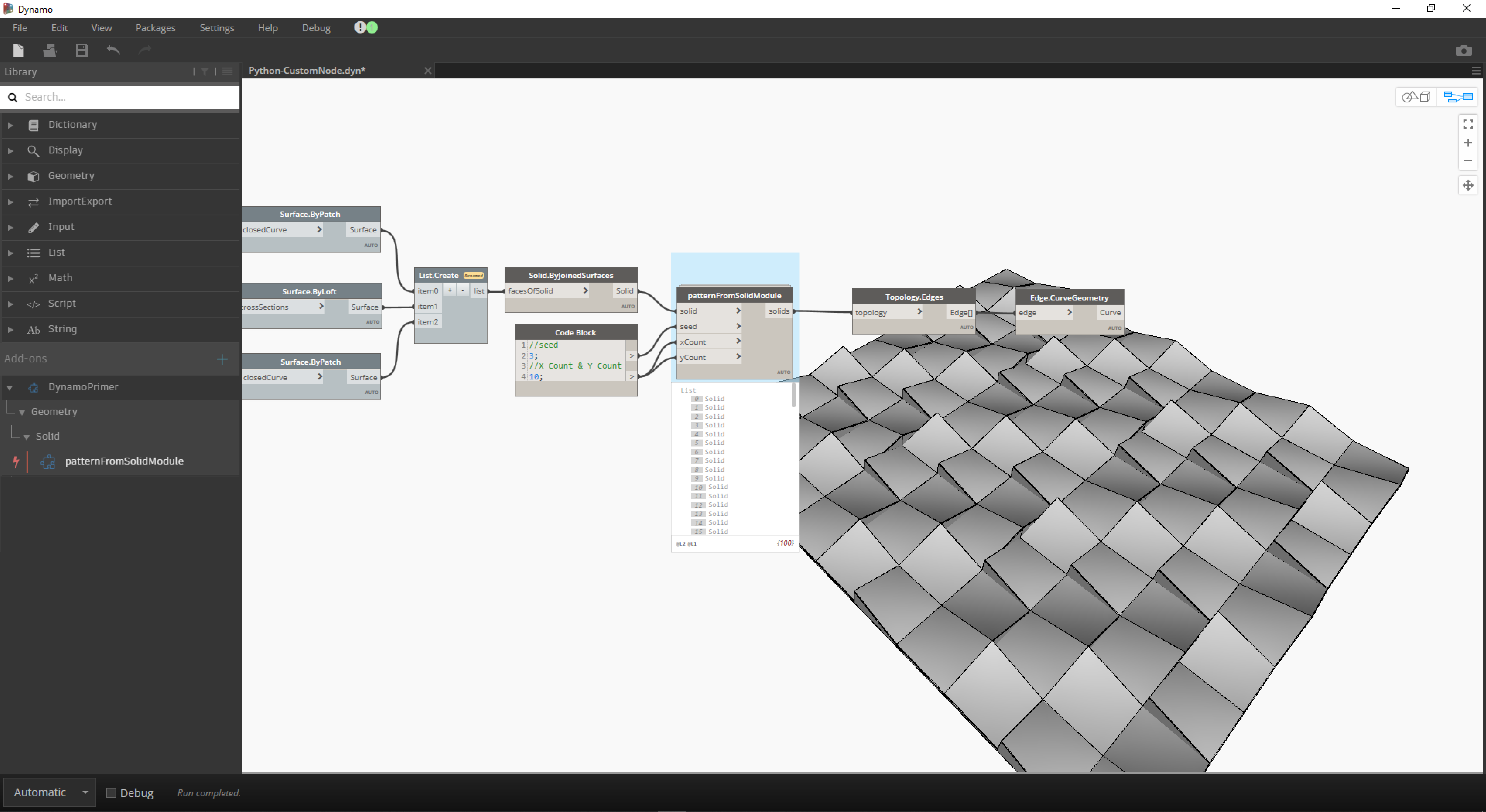## PythonPython 是使用廣泛的程式設計語言，其備受青睞與其語法樣式有很大關係。Python 的可讀性很高，因此較許多其他語言更容易瞭解。Python 支援模組與套件，可嵌入至既有應用程式中。本節中的範例假定您對 Python 有基本瞭解。若要取得有關如何正常使用 Python 的資訊，Python.org 上的「入門」頁面是良好的資源。

### 視覺程式設計與文字程式設計的對比

``````import clr
from Autodesk.DesignScript.Geometry import *

solid = IN
seed = IN
xCount = IN
yCount = IN

solids = []

yDist = solid.BoundingBox.MaxPoint.Y-solid.BoundingBox.MinPoint.Y
xDist = solid.BoundingBox.MaxPoint.X-solid.BoundingBox.MinPoint.X

for i in xRange:
for j in yRange:
fromCoord = solid.ContextCoordinateSystem
toCoord = fromCoord.Rotate(solid.ContextCoordinateSystem.Origin,Vector.ByCoordinates(0,0,1),(90*(i+j%val)))
vec = Vector.ByCoordinates((xDist*i),(yDist*j),0)
toCoord = toCoord.Translate(vec)
solids.append(solid.Transform(fromCoord,toCoord))

OUT = solids
``````

### Python 節點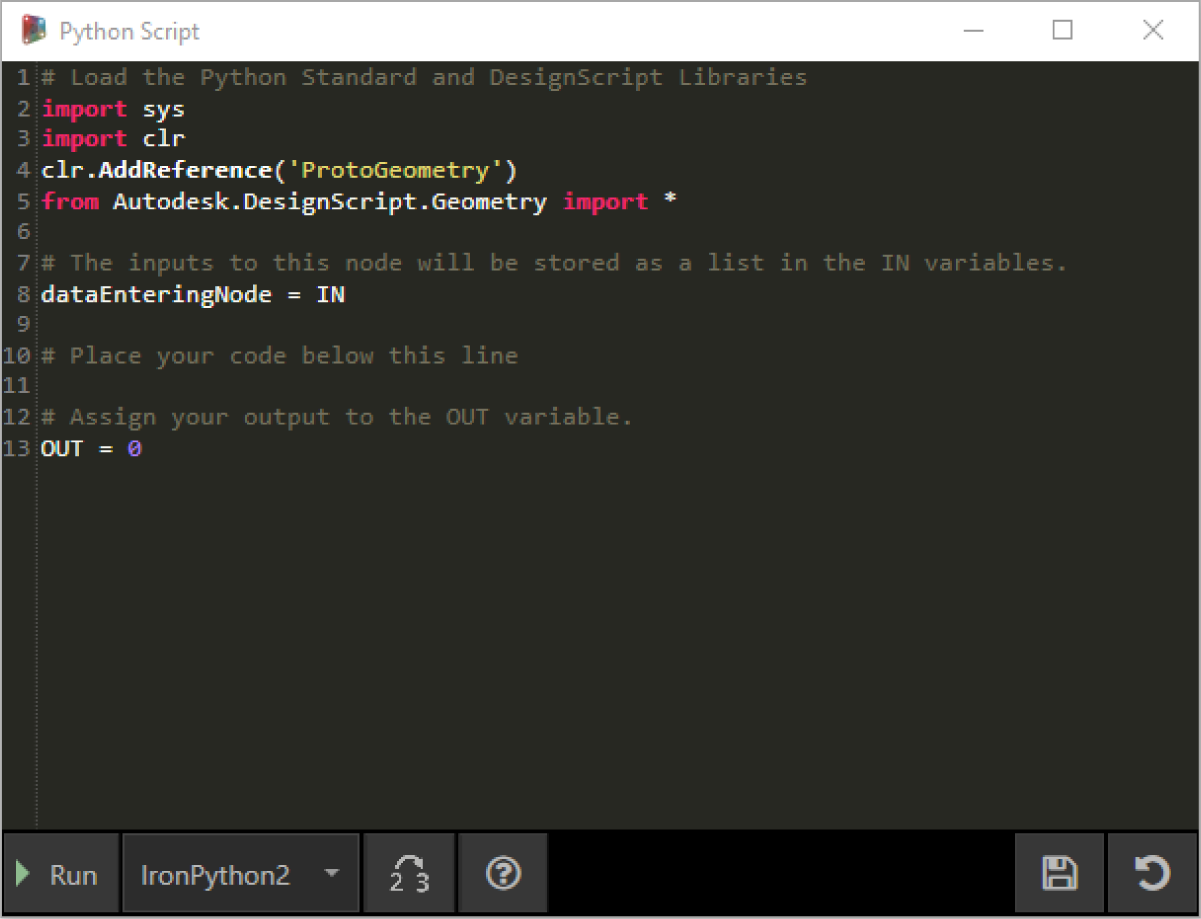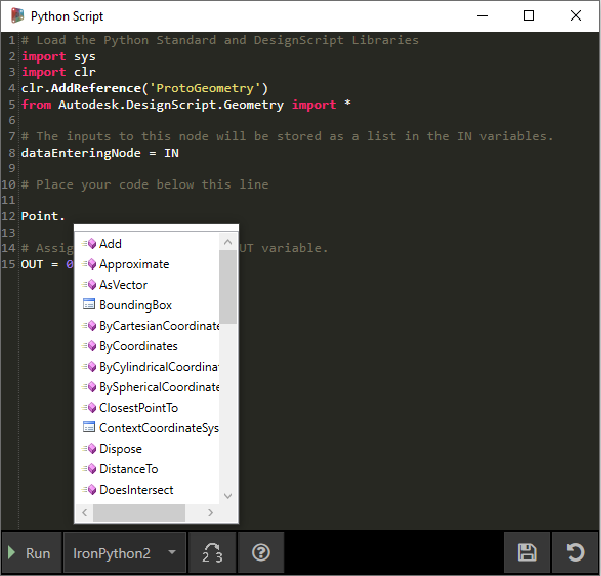### 練習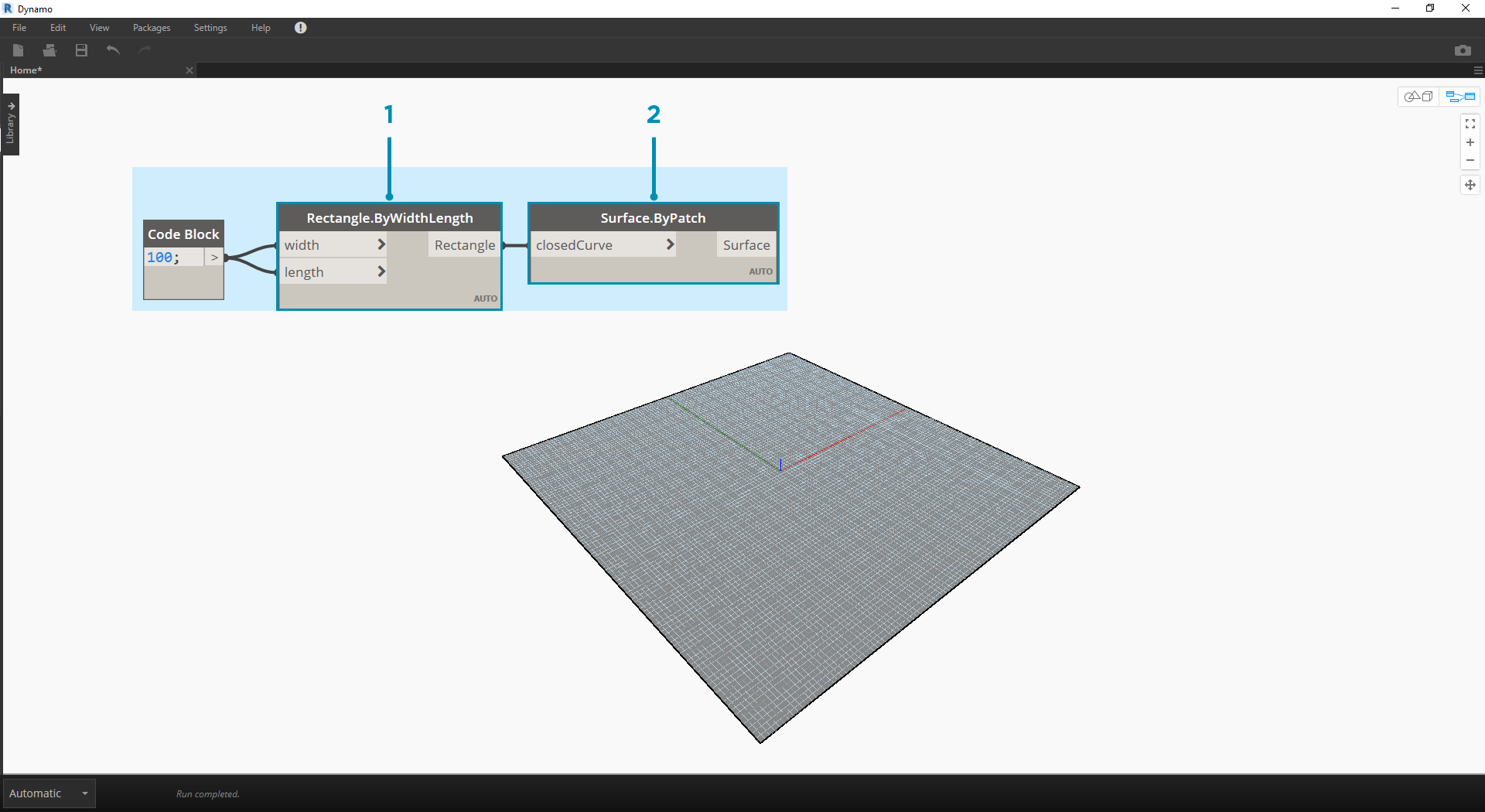1. Rectangle.ByWidthLength：建立將做為實體基準的矩形。
2. Surface.ByPatch：將矩形連接至「closedCurve」輸入以建立底部曲面。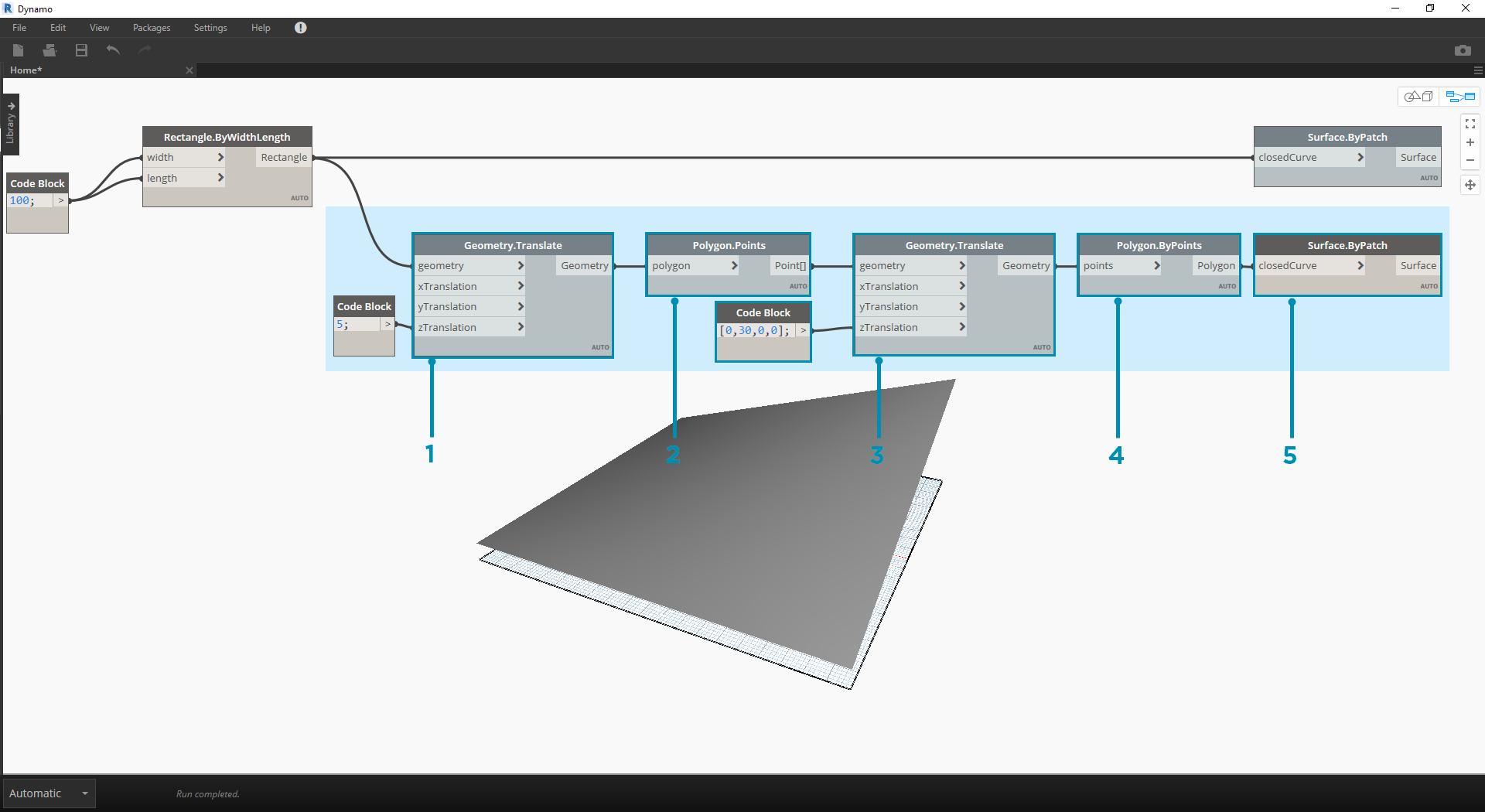1. Geometry.Translate：將矩形連接至「geometry」輸入以將其上移，使用程式碼塊指定實體的基準厚度。
2. Polygon.Points：查詢平移的矩形以萃取角點。
3. Geometry.Translate：使用程式碼塊建立包含四個值 (對應於四個點) 的清單，同時將實體的一個角點上移。
4. Polygon.ByPoints：使用平移的點重新建構頂部多邊形。
5. Surface.ByPatch：連接多邊形以建立頂部曲面。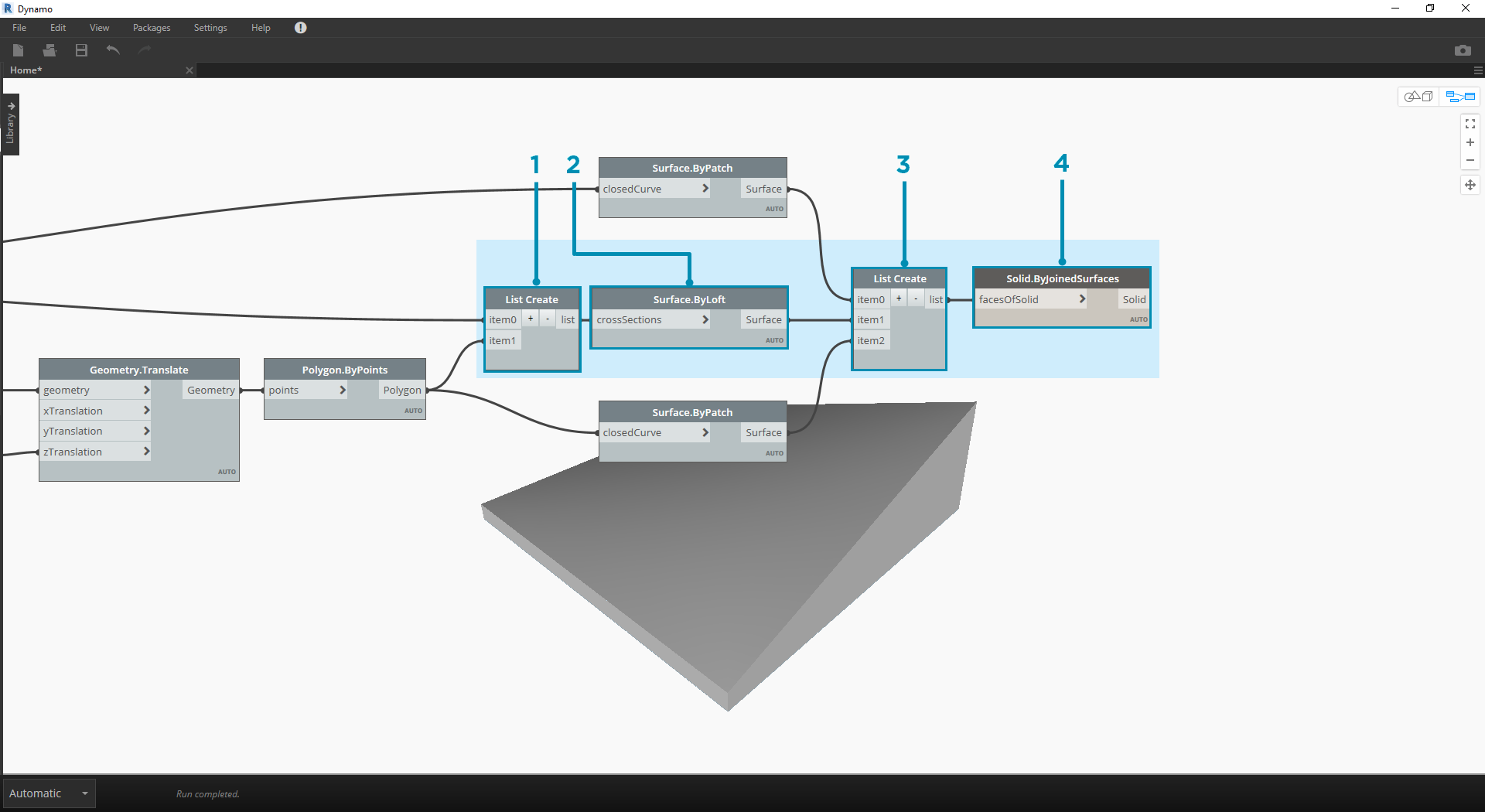1. List.Create：將底部矩形與頂部多邊形連接至索引輸入。
2. Surface.ByLoft：對兩個縱斷面進行斷面混成，以建立實體的側面。
3. List.Create：將頂部、側面與底部的曲面連接至索引輸入，以建立曲面清單。
4. Solid.ByJoinedSurfaces：接合曲面以建立實體模組。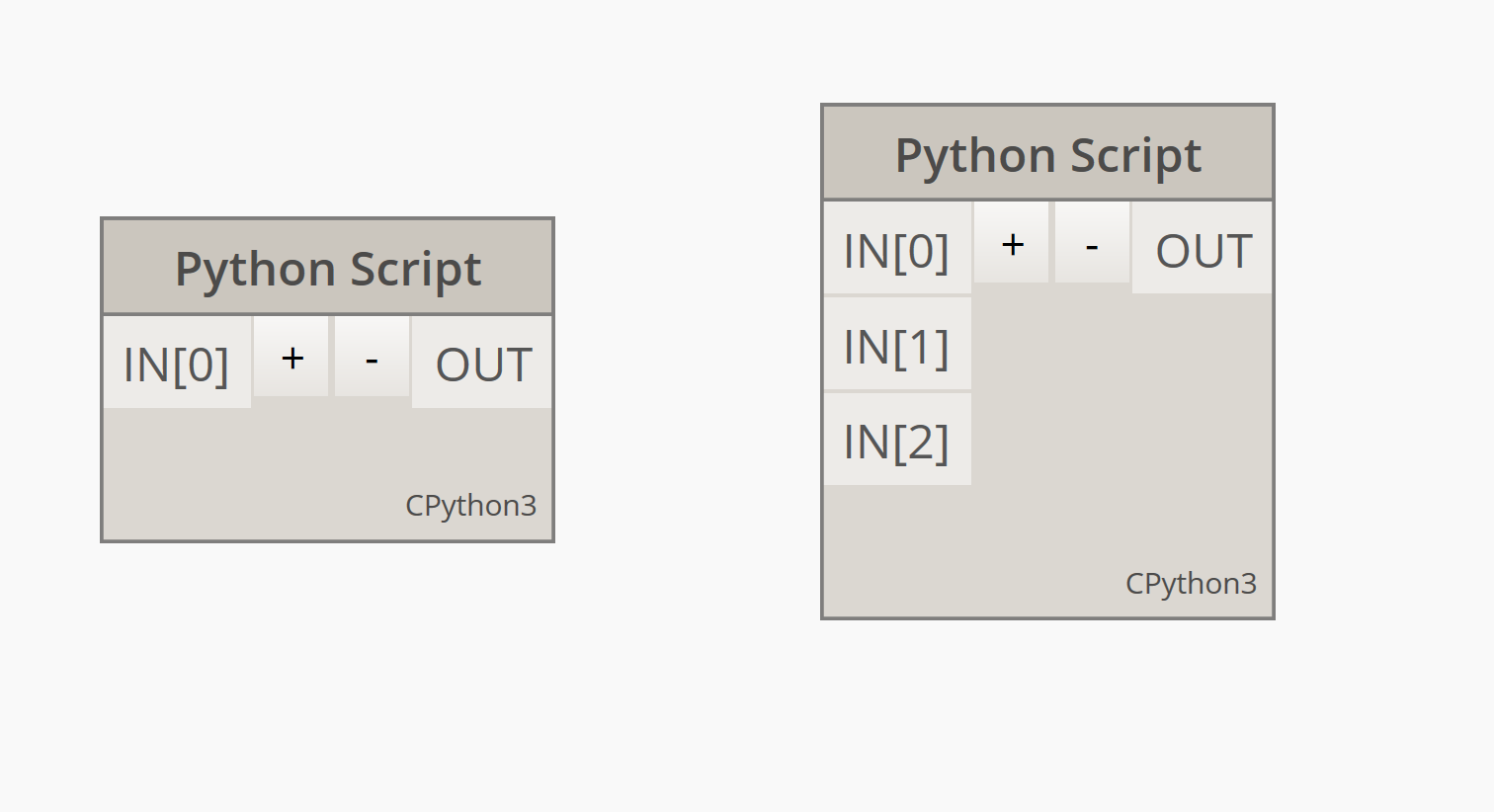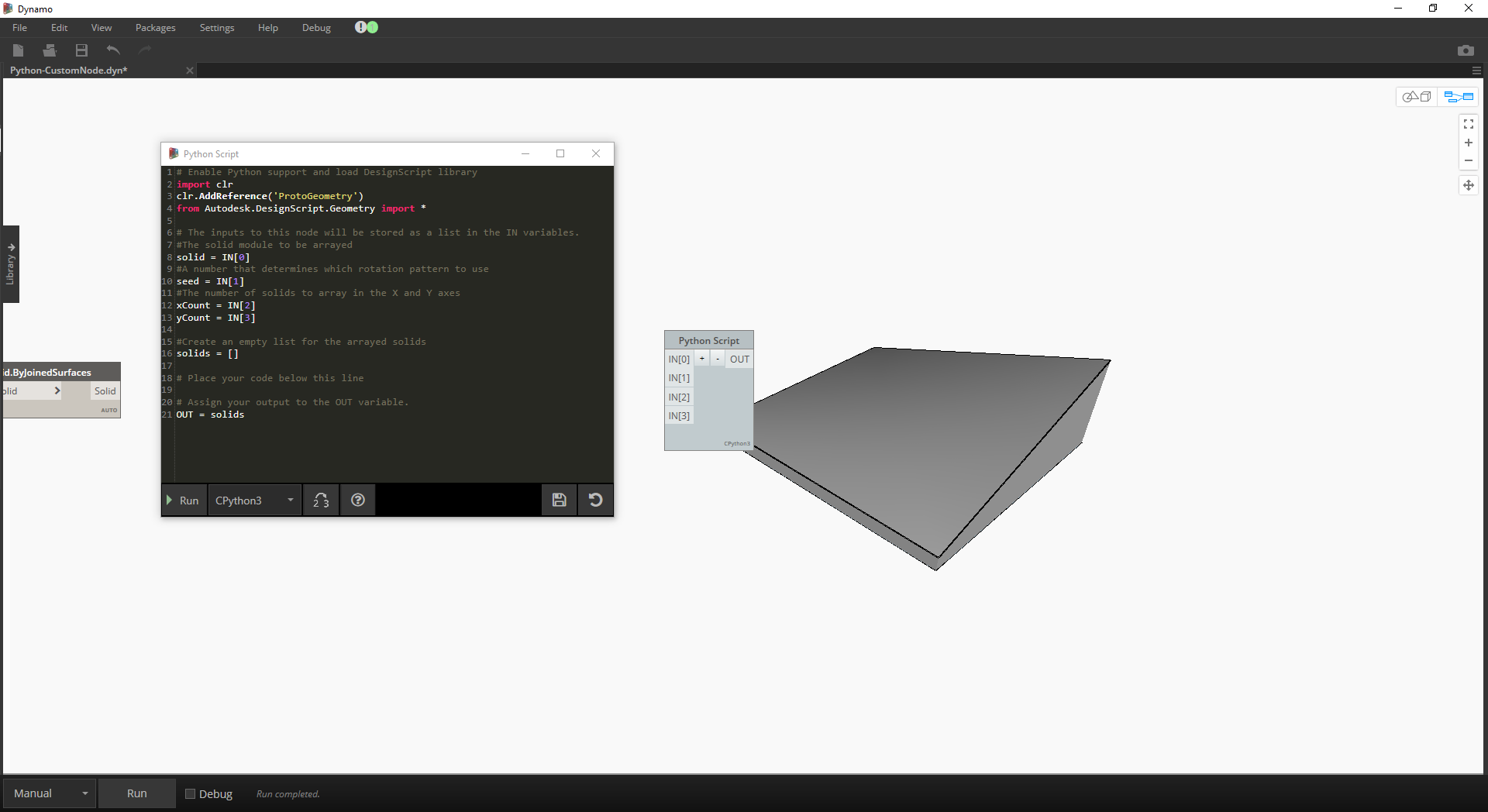``````# Enable Python support and load DesignScript library
import clr
from Autodesk.DesignScript.Geometry import *

# The inputs to this node will be stored as a list in the IN variables.
#The solid module to be arrayed
solid = IN
#A number that determines which rotation pattern to use
seed = IN
#The number of solids to array in the X and Y axes
xCount = IN
yCount = IN

#Create an empty list for the arrayed solids
solids = []

# Place your code below this line

# Assign your output to the OUT variable.
OUT = solids
``````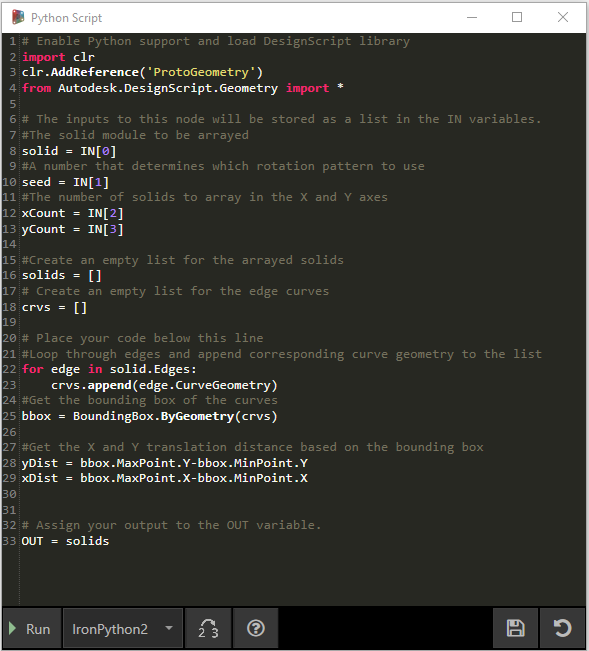``````# Enable Python support and load DesignScript library
import clr
from Autodesk.DesignScript.Geometry import *

# The inputs to this node will be stored as a list in the IN variables.
#The solid module to be arrayed
solid = IN
#A number that determines which rotation pattern to use
seed = IN
#The number of solids to array in the X and Y axes
xCount = IN
yCount = IN

#Create an empty list for the arrayed solids
solids = []
# Create an empty list for the edge curves
crvs = []

# Place your code below this line
#Loop through edges and append corresponding curve geometry to the list
for edge in solid.Edges:
crvs.append(edge.CurveGeometry)
#Get the bounding box of the curves
bbox = BoundingBox.ByGeometry(crvs)

#Get the X and Y translation distance based on the bounding box
yDist = bbox.MaxPoint.Y-bbox.MinPoint.Y
xDist = bbox.MaxPoint.X-bbox.MinPoint.X

# Assign your output to the OUT variable.
OUT = solids
``````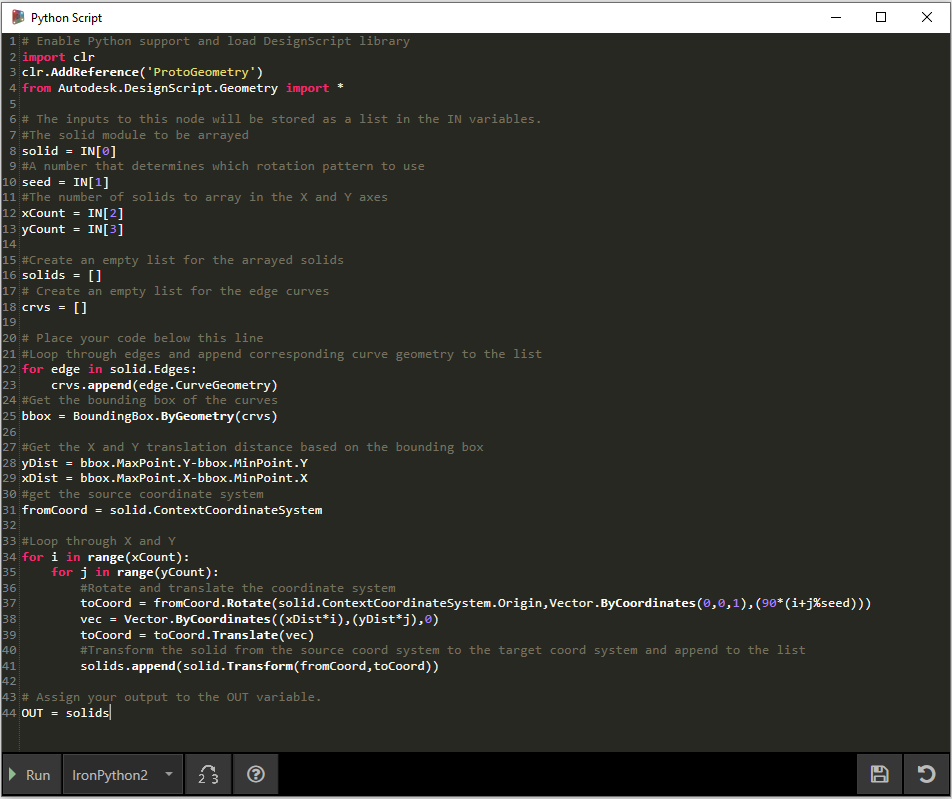``````# Enable Python support and load DesignScript library
import clr
from Autodesk.DesignScript.Geometry import *

# The inputs to this node will be stored as a list in the IN variables.
#The solid module to be arrayed
solid = IN
#A number that determines which rotation pattern to use
seed = IN
#The number of solids to array in the X and Y axes
xCount = IN
yCount = IN

#Create an empty list for the arrayed solids
solids = []
# Create an empty list for the edge curves
crvs = []

# Place your code below this line
#Loop through edges and append corresponding curve geometry to the list
for edge in solid.Edges:
crvs.append(edge.CurveGeometry)
#Get the bounding box of the curves
bbox = BoundingBox.ByGeometry(crvs)

#Get the X and Y translation distance based on the bounding box
yDist = bbox.MaxPoint.Y-bbox.MinPoint.Y
xDist = bbox.MaxPoint.X-bbox.MinPoint.X
#get the source coordinate system
fromCoord = solid.ContextCoordinateSystem

#Loop through X and Y
for i in range(xCount):
for j in range(yCount):
#Rotate and translate the coordinate system
toCoord = fromCoord.Rotate(solid.ContextCoordinateSystem.Origin,Vector.ByCoordinates(0,0,1),(90*(i+j%seed)))
vec = Vector.ByCoordinates((xDist*i),(yDist*j),0)
toCoord = toCoord.Translate(vec)
#Transform the solid from the source coord system to the target coord system and append to the list
solids.append(solid.Transform(fromCoord,toCoord))

# Assign your output to the OUT variable.
OUT = solids
``````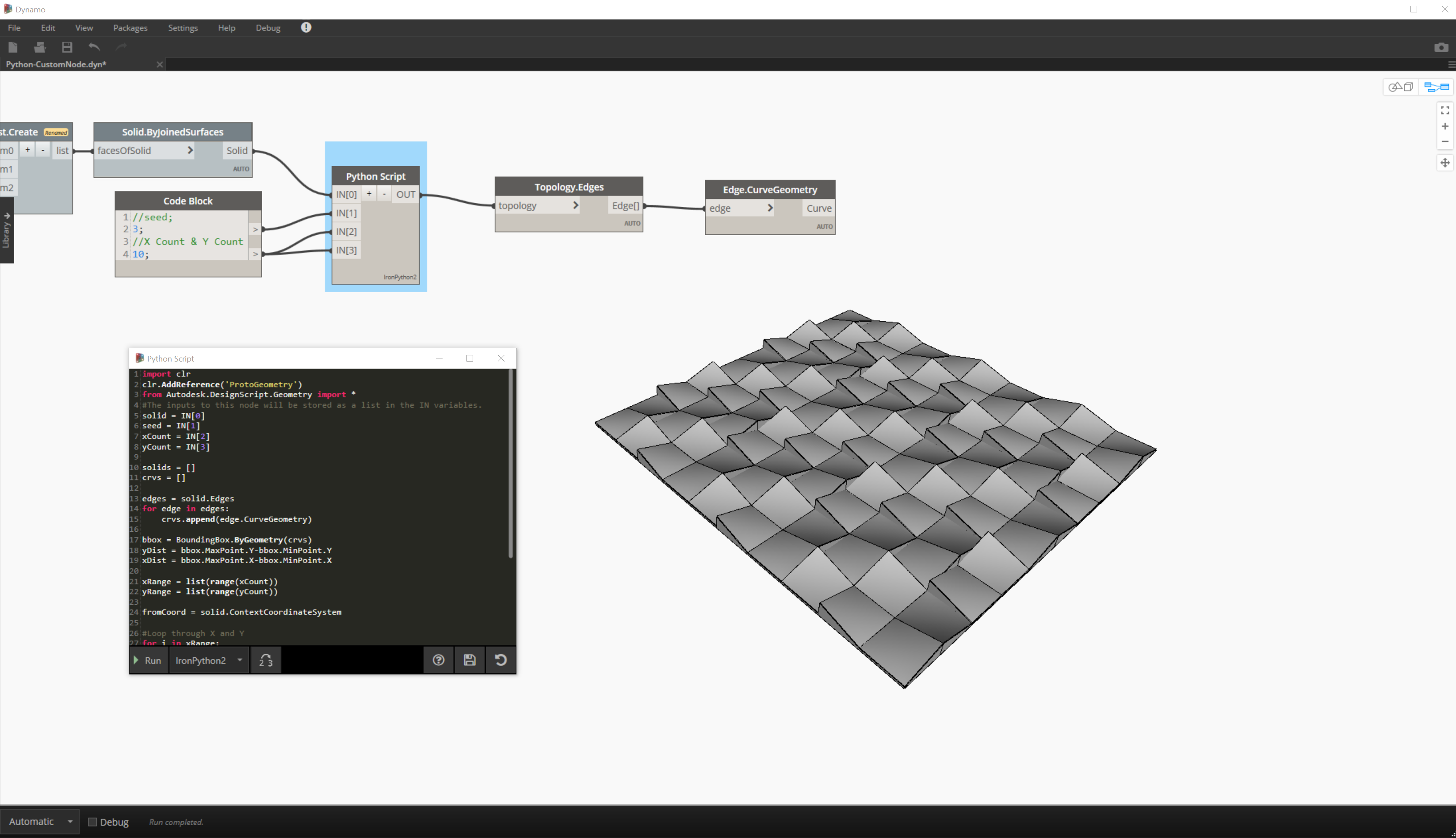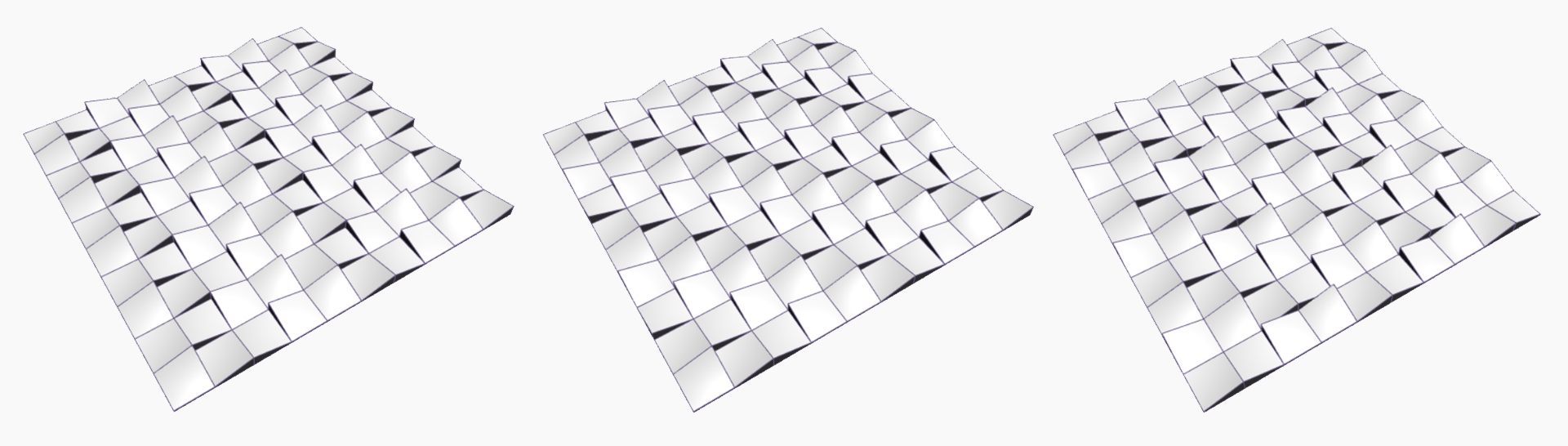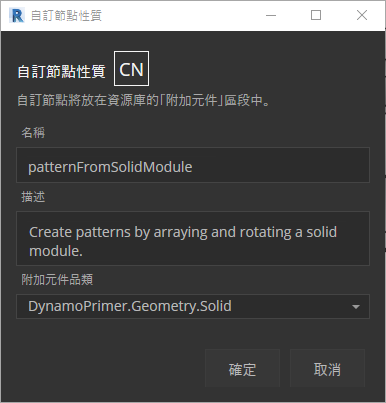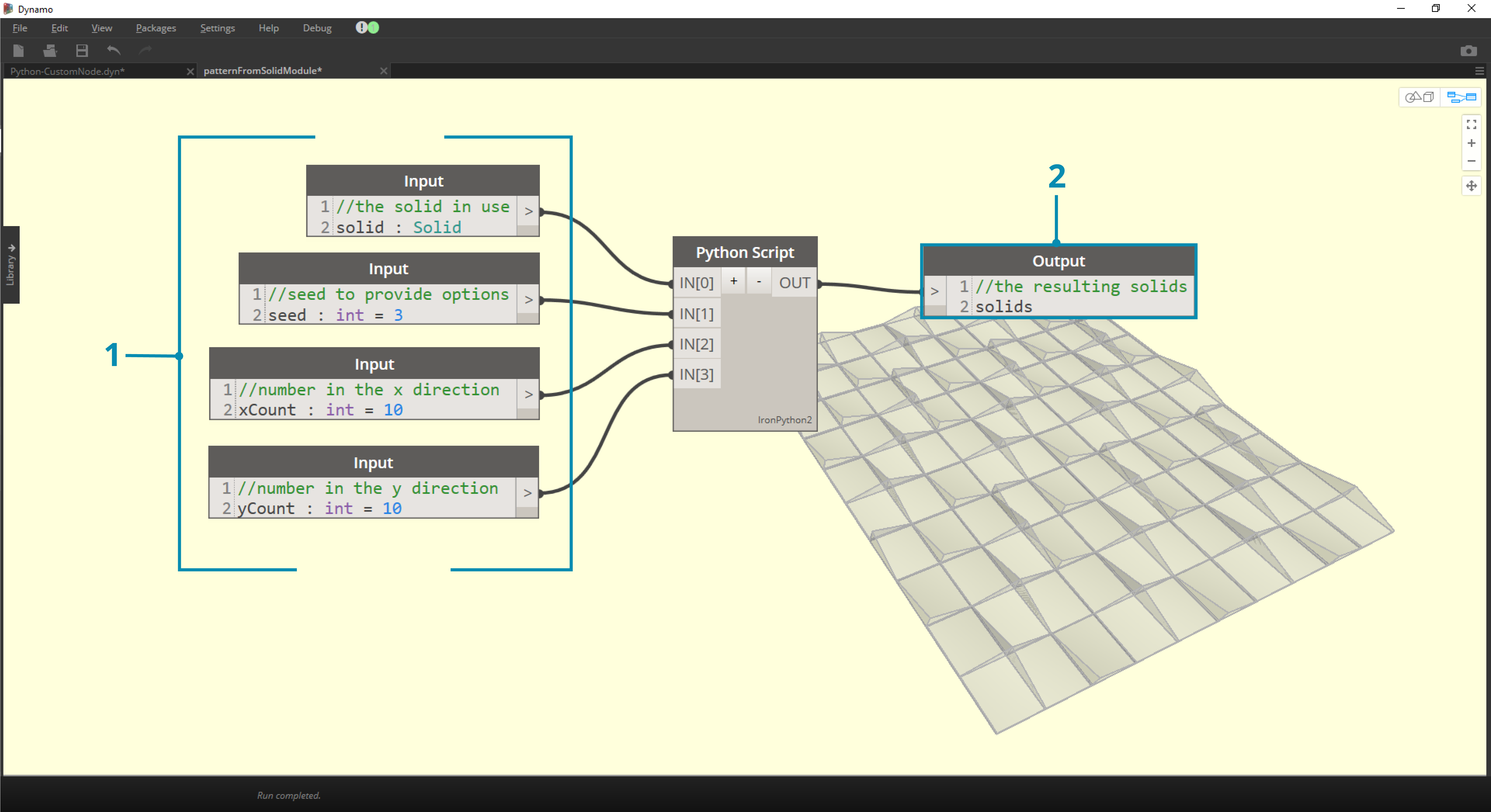1. Input：變更輸入名稱以更具描述性，然後加入資料類型及預設值。
2. Output：變更輸出名稱，將節點另存成 .dyf 檔案。